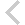FICO Xpress Optimization Examples Repository
 FICO Optimization Community FICO Xpress Optimization HomeFolio - Modelling examples from 'Getting started'

Description
• Chapter 3 Inputting and Solving a Linear Programming problem
• foliolp.mos: modeling and solving a small LP problem
• foliolperr.mos: LP model with syntax errors
• foliolps.mos: LP model using string indices
• Chapter 4 Working with data
• foliodata.mos (data file: folio.dat): data input from file, result output to a file, model parameters
• folioodbc.mos (data files: folio.xls, folio.mdb, folio.sqlite): data input from a spreadsheet or database, result output to a spreadsheet or database, model parameters
• folioexcel.mos (data file: folio.xls): same as folioodbc.mos but with Excel-specific data input and output (Windows only)
• foliosheet.mos (data file: folio.xls): same as folioodbc.mos but with data input and output through generic spreadsheet access
• foliocsv.mos (data file: folio.csv): same as folioodbc.mos but with data input and output through generic spreadsheet access in CSV format
• Chapter 5 Drawing user graphs
• folioloop.mos (data files: folio.dat, foliodev.dat): re-solving with varied parameter settings
• folioloop_graph.mos (data files: folio.dat, foliodev.dat): re-solving with varied parameter settings, graphical solution display
• foliolps_graph.mos: same as foliolps, adding graphical solution display
• Chapter 6 Mixed Integer Programming
• foliomip1.mos (data file: folio.dat): modeling and solving a small MIP problem (binary variables)
• foliomip2.mos (data file: folio.dat): modeling and solving a small MIP problem (semi-continuous variables)
• folioqp.mos (data file: folioqp.dat): modeling and solving a QP and a MIQP problem
• folioqp_graph.mos (data files: folioqp.dat, folioqpgraph.dat): re-solving a QP problem with varied parameter settings, graphical solution display
• folioqc.mos (data file: folioqp.dat): modeling and solving a QCQP and
• foliomiqc.mos (data file: folioqp.dat): modeling and solving a MIQCQP
• Chapter 8 Heuristics
• folioheur.mos (data file: folio.dat): heuristic solution of a MIP problem

Source Files

Data Files

folioloop.mos

```(!******************************************************
Mosel Example Problems
======================

file folioloop.mos
``````````````````
Modeling a small LP problem
to perform portfolio optimization.
-- Re-solving with varied parameter settings --

(c) 2008 Fair Isaac Corporation
author: S.Heipcke, Aug. 2003
*******************************************************!)

model "Portfolio optimization with LP"
uses "mmxprs"

parameters
DATAFILE= "folio.dat"              ! File with problem data
DEVDATA= "foliodev.dat"            ! File with deviation data
MAXVAL = 0.3                       ! Max. investment per share
MINAM = 0.5                        ! Min. investment into N.-American values
end-parameters

declarations
SHARES: set of string              ! Set of shares
RISK: set of string                ! Set of high-risk values among shares
NA: set of string                  ! Set of shares issued in N.-America
RET: array(SHARES) of real         ! Estimated return in investment
DEV: array(SHARES) of real         ! Standard deviation
SOLRET: array(range) of real       ! Solution values (total return)
SOLDEV: array(range) of real       ! Solution values (average deviation)
end-declarations

initializations from DATAFILE
RISK RET NA
end-initializations

initializations from DEVDATA
DEV
end-initializations

declarations
frac: array(SHARES) of mpvar       ! Fraction of capital used per share
Return, Risk: linctr
end-declarations

! Objective: total return
Return:= sum(s in SHARES) RET(s)*frac(s)

! Minimum amount of North-American values
sum(s in NA) frac(s) >= MINAM

! Spend all the capital
sum(s in SHARES) frac(s) = 1

! Upper bounds on the investment per share
forall(s in SHARES) frac(s) <= MAXVAL

! Solve the problem for different limits on high-risk shares
ct:=0
forall(r in 0..20) do
! Limit the percentage of high-risk values
Risk:= sum(s in RISK) frac(s) <= r/20

maximize(Return)                  ! Solve the problem

if (getprobstat = XPRS_OPT) then  ! Save the optimal solution value
ct+=1
SOLRET(ct):= getobjval
SOLDEV(ct):= getsol(sum(s in SHARES) DEV(s)*frac(s))
writeln(r/20, "%: ret ", SOLRET(ct), " dev ", SOLDEV(ct))
else
writeln("No solution for high-risk values <= ", 100*r/20, "%")
end-if
end-do

! Print the data
writeln("Low risk shares:")
forall (s in SHARES - RISK) writeln(s, ": ret ", RET(s), " dev ", DEV(s))

writeln("High risk shares:")
forall (s in RISK) writeln(s, ": ret ", RET(s), " dev ", DEV(s))

end-model

```